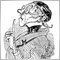# help with code. stop loss varying on each new trade45

Hello, Some help needed on the code below. The EA gives me a different stop loss on each trade yet i want to be fixed at 10pips for the 1hr timeframe. I dont know if it's the sleep function causing it or it's something else.
```input double MaximumRisk   =0.012;
input string InpFileName="decision1hr.csv"; // file name
input string InpDirectoryName="Data"; // directory name
int BarsCount = 0;
double Lots=1.05;
datetime old_bar = 0;
int Ticket;

int open()
{
//--- open the file

{

int    str_size;
int decision;
//--- read data from the file
while(!FileIsEnding(file_handle))
{
//--- find out how many symbols are used for writing the time
//--- print the decision

double SL = 100;
int TP=30000;
RefreshRates();
if(decision==1)
else
if (decision==2)
}
if(Ticket<0)
{
}
else
}

//--- close the file
FileClose(file_handle);
}

int close()
{
for (int i = OrdersTotal() - 1; i >= 0; i--)
{
if(OrderSelect(i,SELECT_BY_POS) == true)
{
int ticket=OrderTicket();
OrderClose(ticket,Lots,Bid,3);
else

}
}
}

int start()
{
if (Bars > BarsCount)
{
close();
Sleep(90000);    // interval
open();
}

BarsCount = Bars;
}
```Moderator
8846

So may be this solves the problem?

```       {Alert("BUY"); Ticket=OrderSend(Symbol(),OP_BUY,Lots,Ask,2,Bid-SL*Point,Bid+TP*Point);}
else
if (decision==2)
```
BTW mql4/mt4 questions belong the the thread at the bottom just for mt4/mql4!45

Carl Schreiber: